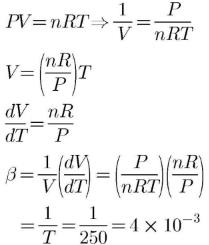Courses

# Test: Boyle's And Charles Law

## 14 Questions MCQ Test Chemistry Class 11 | Test: Boyle's And Charles Law

Description
This mock test of Test: Boyle's And Charles Law for JEE helps you for every JEE entrance exam. This contains 14 Multiple Choice Questions for JEE Test: Boyle's And Charles Law (mcq) to study with solutions a complete question bank. The solved questions answers in this Test: Boyle's And Charles Law quiz give you a good mix of easy questions and tough questions. JEE students definitely take this Test: Boyle's And Charles Law exercise for a better result in the exam. You can find other Test: Boyle's And Charles Law extra questions, long questions & short questions for JEE on EduRev as well by searching above.
QUESTION: 1

### Direction (Q. Nos. 1-4) This section contains 4 multiple choice questions. Each question has four choices (a), (b), (c) and (d), out of which ONLY ONE option is correct. Q. A sample of an ideal gas occupies 20 L under a pressure of 0.5 atm and under isothermal condition. On increasing its pressure to 1.0 atm, its volume will be

Solution:

Since the system is isothermal, so temperature is constant.
According to Boyle's lawP1V1= P2V2
20×0.5=1×V2
10=V2

QUESTION: 2

### A 10.0 cm column of air is trapped by a column of Hg 4.00 cm long in a capillary tube of uniform bore when the tube is held horizontally at 1 atm. Length of the air column when the tube is held vertically with the open end up is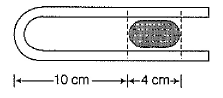Solution:

V1 = 10xcm³
V2 = ?
P1 = 1atm = 760mmHg
P2 = (760 + 4)mmHg
P1V1 = P2V2
V2 = P1V1 / P2
= 760 × 10x / 764
= 9.5x
Therefore,
= 9.5x / x = 9.5

QUESTION: 3

### Volume of an ideal gas is to be decreased by 10% by increase of pressure by x% under isothermal condition. Thus, x is

Solution:

Applying Boyle 's law P1V1=P2V2 as temp is constant.
So let initial pressure =P initial volume =V
Now final volume V2=V-10%of V =9V/10
PV=P2×9V/10 so P2=10P/9
Therefore increment in Pressure=P/9P×100%=100/9

QUESTION: 4

Density of an ideal gas at 298 K and 1.0 atm is found to be 1.25 kg m-3. Density of the gas at 1.5 atm and at 298 K is

Solution:

We know ideal gas equation,
PV=nRT.
n= m(mass)/M(molecular weight).
PV=m/M RT.
PM=m/v RT.
density (d)=m(mass)/v (volume).
PM=dRT.
d=PM/RT.
here, density is directly proportional to pressure and inversely proportional to temperature...
d1/d2=P1 T2 / P2 T1.
1.25/d2= 1×298/1.5×298.
1.25/d2=1/1.5.
d2= 1.25×1.5.
d2=1.875

QUESTION: 5

Direction (Q. Nos. 5-9) This section contains 5 multiple choice questions. Each question has four choices (a), (b), (c) and (d), out of which ONE or  MORE THANT ONE  is correct.

Q. An ideal gas is at 300 K and 1 atm. Then volume remains constant if

Solution:

We know that
pV = nRT
Or V = nRT/p
If we increase both T and P 2 times, only then the volume remains constant.

QUESTION: 6

The pressure of a 1:4 mixture of dihydrogen and dioxygen enclosed in a vessel is one atmosphere. What would be the partial pressure of dioxygen?

Solution:

Let the number of moles of dihydrogen and dioxygen be 1 and 4.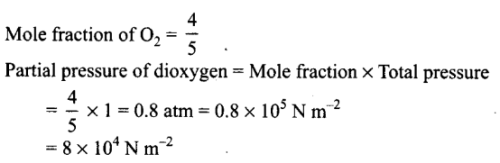*Multiple options can be correct
QUESTION: 7

Select correct matching of laws with their corresponding relations.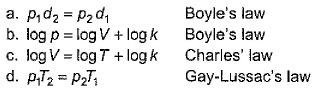Solution: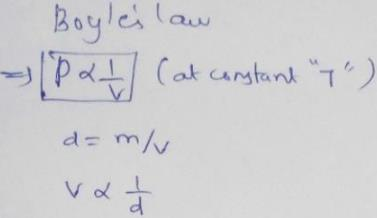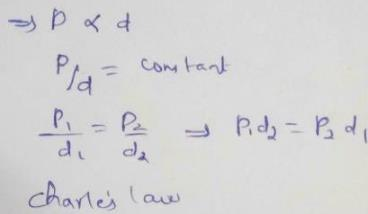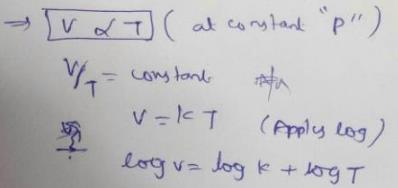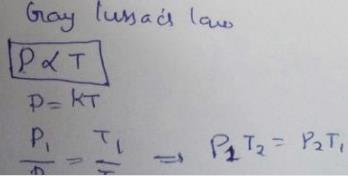QUESTION: 8

Charle's law defines value of 'k' as

Solution:

According to Charles law,at constant pressure, V∝T
Or V/T = k

*Multiple options can be correct
QUESTION: 9

Consider gases confined by a liquid, as shown in figure.
Density of the liquid = d (all values in SI unit)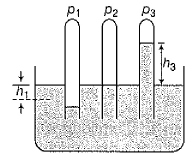Then

Solution:

In this setup
The liquid in tube 1 has got lowered by height h1 which means that the pressure inside the tube is greater than the pressure exerted by the atmosphere. This excess pressure must be equal to the pressure exerted by h1 column of liquid of the same cross section ,therefore
Pressure inside tube 1=atmospheric pressure+h1dg
In tube 2 since the level of water is same as that outside the tube so her
Pressure in tube 2=atmospheric pressure
In tube 3 the liquid has risen by height be which means that pressure inside the tube is less than the pressure exerted by atmospheric pressure .
Atmospheric pressure=pressure in tube+h3dg
Pressure in tube=atmospheric pressure -h3dg

QUESTION: 10

Direction (Q. Nos. 10-11) This section contains a paragraph, wach describing theory, experiments, data etc. three Questions related to paragraph have been given.Each question have only one correct answer among the four given options (a),(b),(c),(d).

For Charles’ law, graphical representation between log V and log T under isobaric condition is shown below for equation V = kT.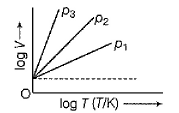Q. Relation between p1, p2 and p3 is

Solution:

From  ideal gas equation, we have
pV = nRT
Or V = nRT/p
Taing log on both sides
log V = log nRT - log p
Or y = mx - c
Let us make a point constant on time axis like this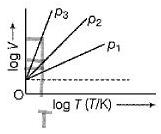Now we have the temperature constant.So to have maximum value of log V, we need to have minimum value of log p. From the graph we can see that we have least pressure for p3 . So the order will be p3<p2<p1.

QUESTION: 11

For Charles’ law, graphical representation between log V and log T under isobaric condition is shown below for equation V = kTQ. Volume V = constant k, when

Solution:

-272deg.
For C = 1 K
V / 1 = K
V = K

QUESTION: 12

Direction (Q. Nos. 12) Choice the correct combination of elements and column I and coloumn II  are given as option (a), (b), (c) and (d), out of which ONE option is correct.

Q.
G
raphicaily Boyle’s law can be represented by various options depending on coordinates. Match the graphs in Column t with their coordinates in Column II and select the correct alternate from the codes given beiow.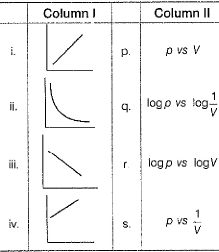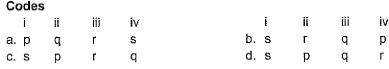Solution:

From Boyle’s law;
p ∝ 1/V ⇒ Graph i (Straight line passing through origin)
Or pV = Constant ⇒ Graph ii (rectangular hyperbola)
Taking log on both side;
log(pV) = logK
logp + logV = k’
logp = -logV = k’ (y = -mx+c) ⇒ Graph iii
log p = log V-1 + k’
Logp = log(1/V) + k’ ⇒ Graph iv
So option c is correct

*Answer can only contain numeric values
QUESTION: 13

Direction (Q. Nos. 13 and 14) This section contains 2 questions. when worked out will result in an integer from 0 to 9 (both inclusive).

Isothermally compressibility of an ideal gas is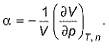Q.
What is the value of a at 0.2 bar?

Solution:

α = -1/V(dV/dP)T
pV = RT;
V = RT/p
dV/dp = -RT/p2
α = -1/(RT/p)×(-RT/p2)
α = 1/p
Or α =  (since p = 0.2)

*Answer can only contain numeric values
QUESTION: 14

Cubic expansion coefficient (β) for an ideal gas is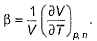Q.
Its value at 250 K is x * 10-3 K -1. What is value of x?

Solution: